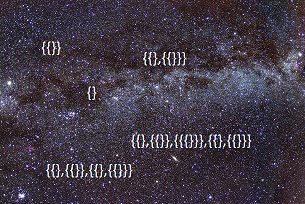Home > Number theory

# Set theory

Set theory is a branch of mathematics that deals with sets. A set simply consists of some elements. These elements can be anything. In fact, they can even be sets! However, you cannot just allow all sets as this would create paradoxes. An example is Russell's paradox, also known as the barber paradox. Imagine there is a set (barber) such that it contains (shaves) all sets (inhabitants of the town) that do not contain themselves. Does the set contain itself? If it does, it doesn't. However, if it doesn't, it does.

This is the problem with what is called naive set theory. To make it rigorous, axiomatic set theory states that sets can only be constructed using axioms and sets already constructed. The most common of these is ZFC, which stands for Zermelo-Fraenkel set theory with the axiom of choice. There are many axioms. Here are the informal definitions.

1: If two sets have the same elements, they are the same set. (For example, the set {a, b, c} is the same as the set{c, b, a})

2: Every set has an element disjoint to itself. (This means that the set and its element have no elements in common. For example, the set {{a, b}, {{a, b}}} has the element {a, b} which has no elements in common with the original set as neither a nor b are actually elements of the original set)

3: For all sets and functions, there is a set that contains all images of the elements in the original set. (For example, let the set be {{a, b}, {c, d}, {e, f, g}} and let the function be adding h to a set. This axiom states that the set {{a, b, h}, {c, d, h}, {e, f, g,h}} exists)

4: For any two sets, there is a set with both as elements. (For example, let the sets be {a, b} and {c, d}. The axiom states that the set {{a, b}, {c, d}} exists)

5: Given any two sets, the union of the sets is a set. (For example, let the sets be {a, b} and {c, d}. The axiom states that the set {a, b, c, d} exists. This is not to be confused with Axiom 4)

6: There is a set with infinitely many elements. (For example, the set {1, 2, 3, 4...}

7: Given any set, there is a set of all subsets of that set. {For example, given the set {a, b, c}, the axiom states that the set {{}, {a}, {b}, {c}, {a, b}, {a, c}, {b, c}, {a, b, c}} exists.

8: Given some sets, you can pick an element from each to make a new set. (This is the Axiom of Choice. For example, given the sets {a, b}, {c, d}, {e, f} this states that, for example, the set {a, c, f} or any other containing one element from each exists. This seems obvious but is important and somewhat hard to grasp for infinitely many sets)

Some basic sets can be constructed. An ordinal can be thought of as the set of previous ordinals. Zero is the empty set {}. From there, we define 1 as {{}}, 2 as {{},{{}}}, 3 as {{},{{}},{{},{{}}}}, and so on. However, this is not all. We can actually define any possible set, as long as it is not contained in itself, its elements, its elements' elements, and so on. We can recursively define sets starting at the empty set and then taking all sets containing previously-defined sets as elements.

There is also a Von Neumann universe of sets. This is Va, where a is an ordinal. V0 has no elements. Each universe has as its elements all the sets of elements of the preceding one. Thus V1 has {} as an element and V2 has {} and {{}}. V3 has 4 elements, V4 has 16, V5 has 65536, and V6 has more elements than the number of particles in the observable (physical) universe. In general, if Vx has y elements, Vx+1 has 2y elements. We can continue into ordinals. Vω has aleph-0 elements. Lastly, V, the Von Neumann universe, has all sets of elements in all universes. For any universe, there is a set in V that has all elements in the universe. However, there is no set that contains all elements of V. Such a set would create paradoxes such as Russell's paradox. Thus V itself is not a set. However, V has other properties. V has as elements all sets defined by the recursive method mentioned above.The "Von Neumann Universe"

•Coming soon